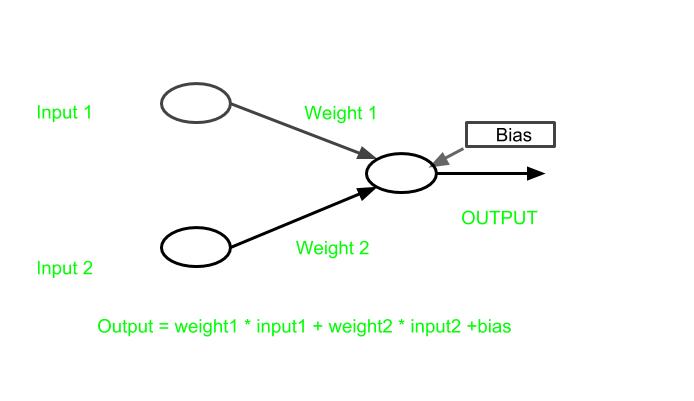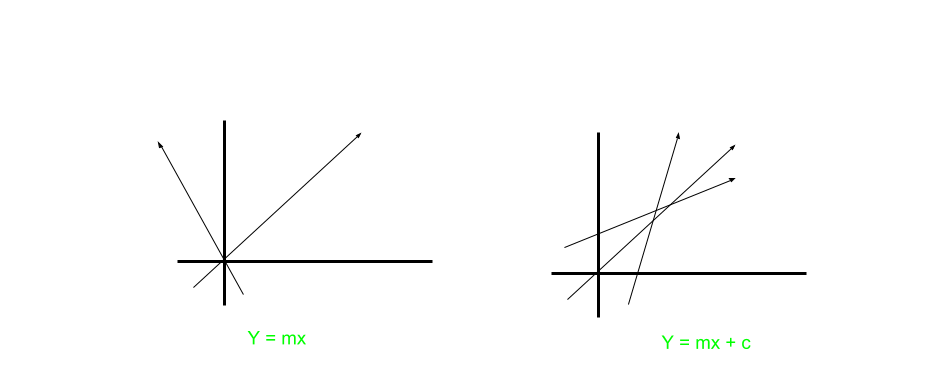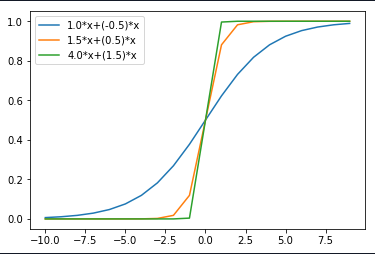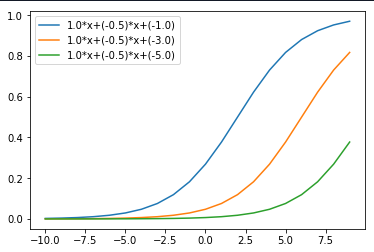×

Search anything:

# Bias in Machine learning

#### Machine Learning (ML) biasGet this book -> Problems on Array: For Interviews and Competitive Programming

When reading up on artificial neural networks, you may have come across the term “bias.” It’s sometimes just referred to as bias. Other times you may see it referenced as bias nodes, bias neurons, or bias units within a neural network. We’re going to break this bias down and see what it’s all about.

We will first start out by discussing the most obvious question of, well, what is bias in an artificial neural network/ machine learning in general? We will then see, within a network, how bias is implemented. Then, to hit the point home, we will explore a simple example to illustrate the impact that bias has when introduced to a neural network.

# What is bias?

Bias is an constant parameter in the Neural Network which is used in adjusting the output. Therefore Bias is a additional parameter which helps the model so that it can perfectly fit for the given data.

The processing can be denoted as :

``````output  =  sum (weights * inputs) + bias
``````# Implementation

In the neural network, we have been given input x and for that input, we need to predict the output y. Here, we create a model (mx + c), which predicts the output.

In below figure,

``````y = mx+c,
m  =  weight
c  =  bias
``````While training the model our main aim is to find the appropriate values of the constants m and c.Let's consider the first case where we have the model as y = mx instead of y = mx + c.

Here, the model is having a limitation in training as many times for the given data, it is impossible for the algorithm to fit the model so that it passes through the origin.

So what we need now is an algorithm that fits the model almost everytime we train our data.

So let's give some freedom to the algorithm by changing the model as mx + c instead of mx, so that the model can find a line which fits the given data.
Now, it is having the full freedom to train itself and find a model which fits the best for the given data.

Also with the introduction of bias, the model will become more flexible.
In other words, Bias is a constant which gives freedom to perform best.

# Example

Now Suppose we have an activation function actv() which gets triggered on input greater than 0.

Now,

``````input1 = 1
weight1 = 2
input2 = 2
weight2 = 2
``````

so,

``````output  =  (input1 * weight1 + input2 * weight2)
output  =  6
``````

let's assume,

``````actv(output)  =  1
``````

Now a bias is introduced in output as

``````bias  =  -6
``````

so our output becomes,

``````mx+c = 6+(-6)= 0.
actv(0)  =  0
``````

so activation function will not trigger.

Change in weight
Here in graph below,As it can be seen that when weight W1 changed from 1.0 to 4.0 and weight W2 changed from -0.5 to 1.5, the steepness is increasing.

Therefore it can be concluded that more is the weight more the activation function will trigger.

Change in bias
Here in graph below,When Bias changed from -1.0 to -5.0, It led to the increase in the value of triggering activation function.

Therefore it can be inferred that from above graph that
bias helps in controlling the value at which activation function will trigger.

# End Notes:

Here we saw about the Bias in neural network and its implementation through an example and learnt about its importance.#### Prashant Anand

Bachelor of Technology (2016 to 2020) in Electronics and Communications Engineering at Reva University, Bangalore | Intern at OpenGenus

Bias in Machine learning